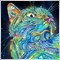• Visão global
• Comentários (1)
• Discussão (1)

# Forecast

We present our trend indicator Forecast. The indicator is designed for identifying entry and exit points, and can be used alone or in combination with other technical analysis tools.

Four parameters are required for the indicator:

• BarSearch - determines the number of candlesticks in history for analysis
• Forecast - sets the range of forecasting in candlesticks
• Depth - sets the depth of analysis
• Lag - defines the delay in candlesticks before recalculating the indicator

Here are the details of how the indicator works.

First the indicator defines the trend of the currency pair movement. Using the BarSearch parameter, the indicator analyzes the specified number of candlesticks, determines the regression line and draws the regression line and channel in the chart window.

Then, based on the analysis of the closing prices of candlesticks from the regression line, the indicator calculates the formula of similarity for the price movement using a polynomial with a degree specified by Depth. When you specify a value for Depth, pay attention to the following:

• a too small value of this parameter will result in a function with a large deviation from the actual price, which will give greater error;
• a too large value of this parameter will result in a higher load on the PC for the indicator calculation and, as a consequence, in longer calculations. Another aspect of the high value of this parameter is that with a more accurate likeness of the currency pair the function will repeat various "noises" caused by the influence of external factors on the market.

Next, based on the calculated polynomial, the indicator constructs a of the price movement in history, which continues on the predicted number of candlesticks set in the Forecast parameter. It also draws a channel of the average deviation of the actual close prices of candlesticks from the calculated line.

Using the Lag parameter (delay before indicator recalculation) allows the trader to assess the accuracy of the estimated forecast of the currency pair movement based on how actual candlesticks fit the theoretical line.

All the parameters of the indicator can be changed in a wide range and are not limited by the program. However, limits should be set base on the capabilities of the hardware and the common sense. It should be noted that the BarSearch parameter should not exceed the number of candlesticks in the MetaTrader 4 history, and a too high value of Forecast will lead to less accurate predictions.430
2015.03.20 16:50

то что доктор прописал